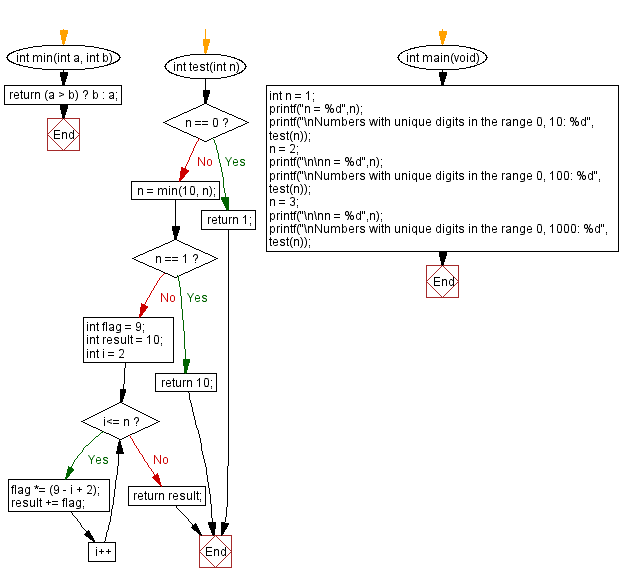﻿ C - Count all the numbers with unique digits in a range

# C Programming: Count all the numbers with unique digits in a range

## C Programming Mathematics: Exercise-30 with Solution

Write a C program that accepts a number (n) and counts all numbers with unique digits of length x within a specified range.

Range: 0 <= x < 10n

Example:
When n = 1, numbers with unique digits (10) between 0 and 9 are 0, 1, 2, 3, 4, 5, 6, 7, 8, 9
When n = 2, numbers with unique digits (91) between 0 and 100 are 0,1, 2, 3, 4, 5, 6, 7, 8, 9, 10, 12, 13, 14, 15 …..99 except 11, 22, 33, 44, 55, 66, 77, 88 and 99.

Test Data:
(1) -> 10
(2) -> 91

Sample Solution:

C Code:

``````#include <stdio.h>

int min(int a, int b){
return (a > b) ? b : a;
}
int test(int n) {
if(n == 0)
return 1;
n = min(10, n);
if(n == 1)
return 10;
int flag = 9;
int result = 10;
for(int i = 2; i<= n; i++){
flag *= (9 - i + 2);
result += flag;
}
return result;
}

int main(void) {
int n = 1;
printf("n = %d",n);
printf("\nNumbers with unique digits in the range 0, 10: %d", test(n));
n = 2;
printf("\n\nn = %d",n);
printf("\nNumbers with unique digits in the range 0, 100: %d", test(n));
n = 3;
printf("\n\nn = %d",n);
printf("\nNumbers with unique digits in the range 0, 1000: %d", test(n));
}
```
```

Sample Output:

```n = 1
Numbers with unique digits in the range 0, 10: 10

n = 2
Numbers with unique digits in the range 0, 100: 91

n = 3
Numbers with unique digits in the range 0, 1000: 739
```

Flowchart:C Programming Code Editor:

Improve this sample solution and post your code through Disqus.

What is the difficulty level of this exercise?

Test your Programming skills with w3resource's quiz.

﻿